# 支持向量机SVM（Support Vector Machine）

## 一、支持向量机的原理

Support Vector Machine。支持向量机，其含义是通过支持向量运算的分类器。其中“机”的意思是机器，可以理解为分类器。 那么什么是支持向量呢？在求解的过程中，会发现只根据部分数据就可以确定分类器，这些数据称为支持向量。 见下图，在一个二维环境中，其中点R，S，G点和其它靠近中间黑线的点可以看作为支持向量，它们可以决定分类器，也就是黑线的具体参数。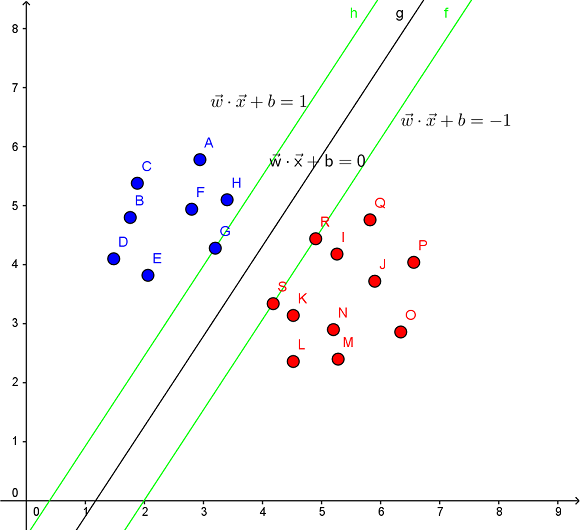• 线性分类

• 非线性分类

SVM的一个优势是支持非线性分类。它结合使用拉格朗日乘子法和KKT条件，以及核函数可以产生非线性分类器。

SVM的目的是要找到一个线性分类的最佳超平面 f(x)=xw+b=0。求 w 和 b。

SVM的解决问题的思路是找到离超平面的最近点，通过其约束条件求出最优解。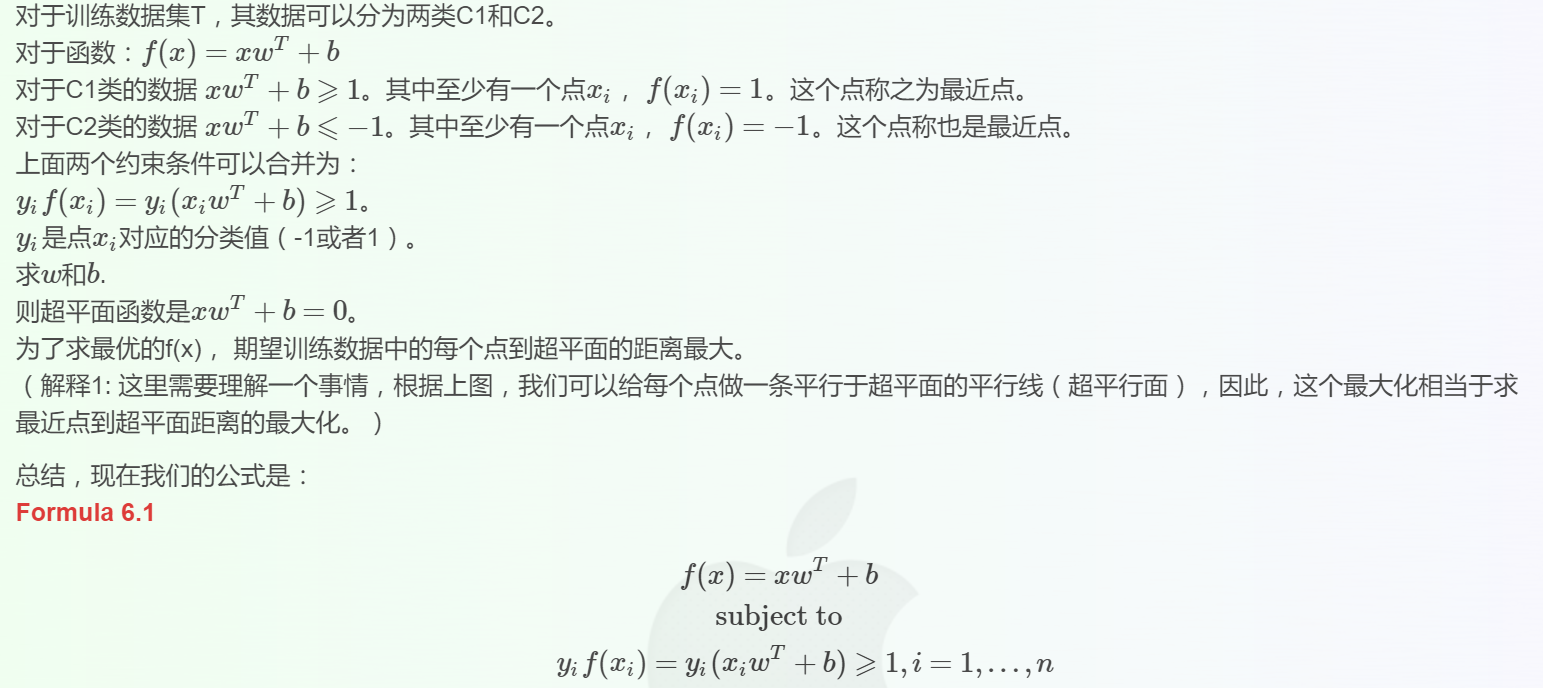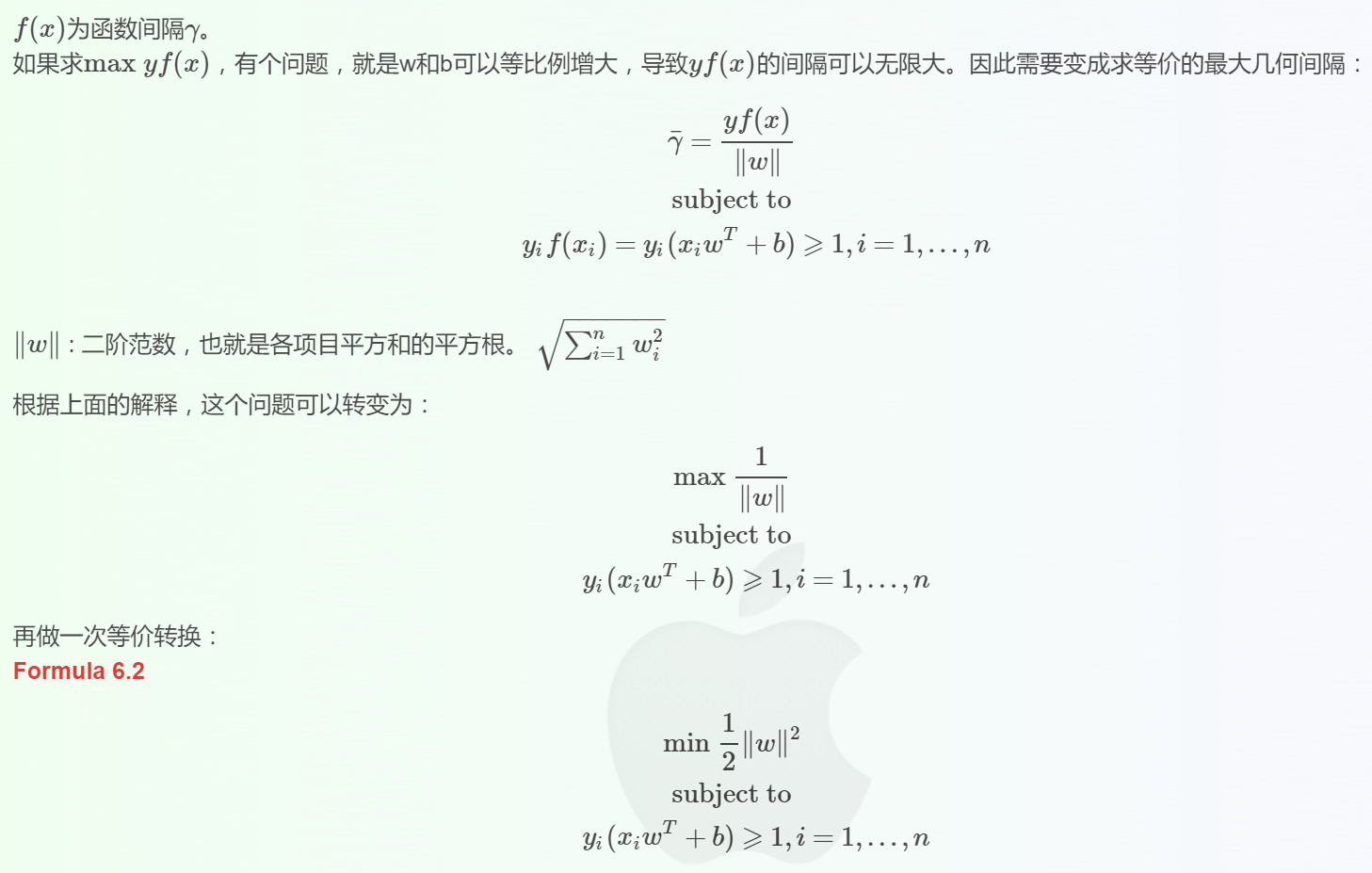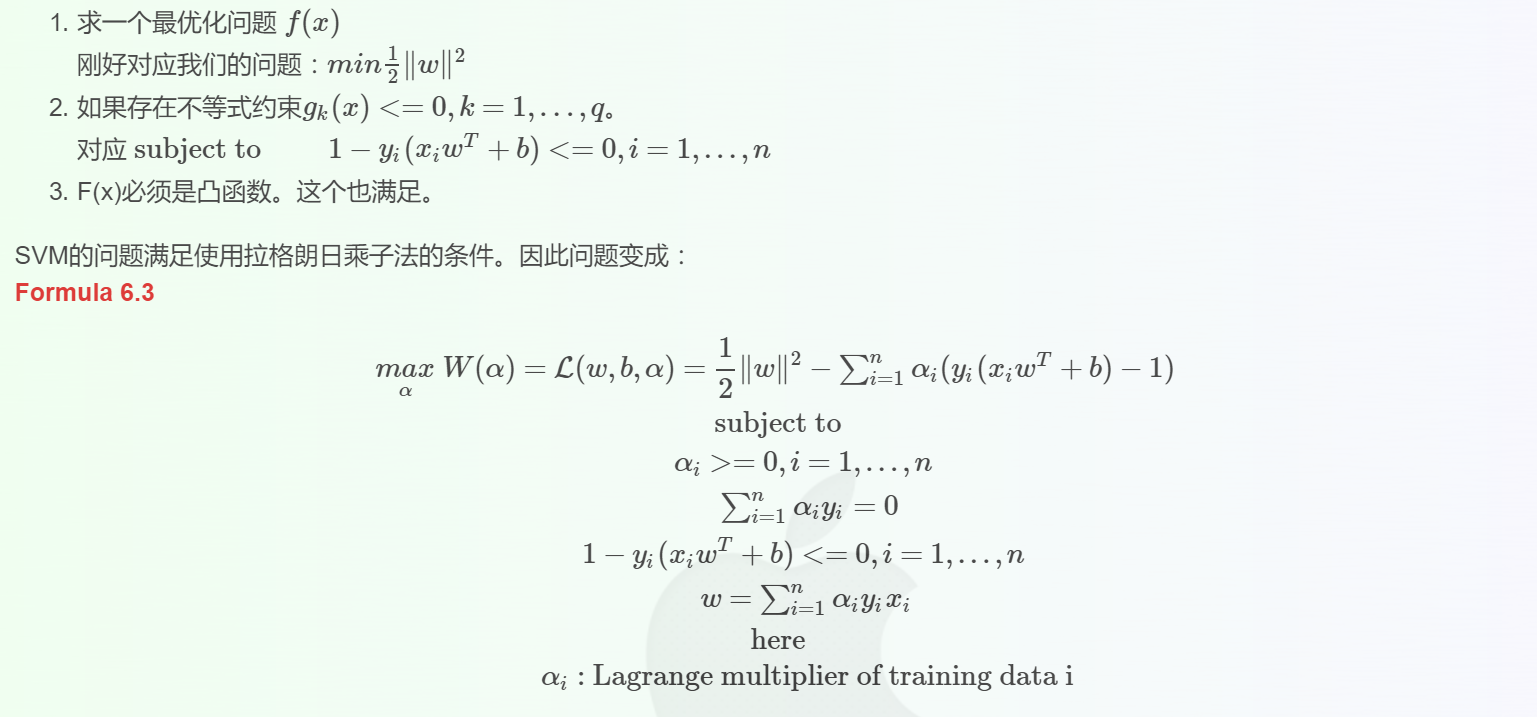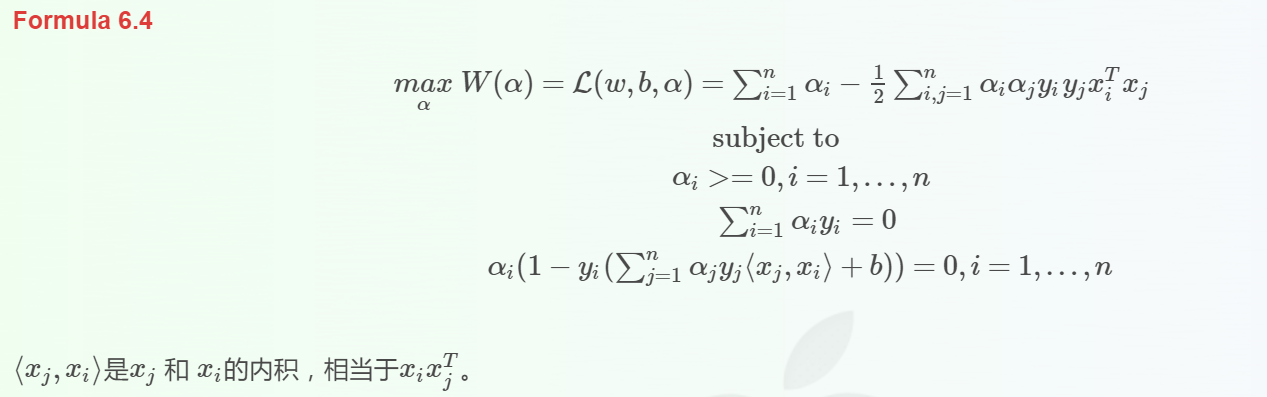### SVC参数解释

• （1）C: 目标函数的惩罚系数C，用来平衡分类间隔margin和错分样本的，default C = 1.0；
• （2）kernel：参数选择有RBF, Linear, Poly, Sigmoid，precomputed或者自定义一个核函数, 默认的是"RBF"，即径向基核，也就是高斯核函数；而Linear指的是线性核函数，Poly指的是多项式核，Sigmoid指的是双曲正切函数tanh核。  在这里需要具体讲一下各种核函数怎么使用： RBF核：高斯核函数就是在属性空间中找到一些点，这些点可以是也可以不是样本点，把这些点当做base，以这些base为圆心向外扩展，扩展半径即为带宽，即可划分数据。换句话说，在属性空间中找到一些超圆，用这些超圆来判定正反类。   线性核和多项式核：这两种核的作用也是首先在属性空间中找到一些点，把这些点当做base，核函数的作用就是找与该点距离和角度满足某种关系的样本点。当样本点与该点的夹角近乎垂直时，两个样本的欧式长度必须非常长才能保证满足线性核函数大于0；而当样本点与base点的方向相同时，长度就不必很长；而当方向相反时，核函数值就是负的，被判为反类。即，它在空间上划分出一个梭形，按照梭形来进行正反类划分。   Sigmoid核：同样地是定义一些base，核函数就是将线性核函数经过一个tanh函数进行处理，把值域限制在了-1到1上。   总之，都是在定义距离，大于该距离，判为正，小于该距离，判为负。至于选择哪一种核函数，要根据具体的样本分布情况来确定。
• （3）degree：degree决定了多项式的最高次幂；
• （4）gamma：核函数的系数('Poly', 'RBF' and 'Sigmoid'), 默认是gamma = 1 / n_features，即核函数的带宽，超圆的半径;
• （5）coef0：核函数中的独立项，'RBF' and 'Poly'有效，即加在这两种核函数后面的bias值，次幂为0的项，默认是0.0，即认为多项式的核的常数项为0；
• （6）probablity: 布尔取值，默认是false，指的是预测判定的时候是否采用概率估计；
• （7）shrinking：是否进行启发式；
• （8）tol（default = 1e - 3）: svm结束标准的精度，即容忍1000分类里出现一个错误;
• （9）cache_size: 制定训练所需要的内存（以MB为单位）；
• （10）class_weight: 正类和反类的样本数量是不一样的，这里就会出现类别不平衡问题，该参数就是指每个类所占据的权重，默认为1，即默认正类样本数量和反类一样多，也可以用一个字典dict指定每个类的权值，或者选择默认的参数balanced，指按照每个类中样本数量的比例自动分配权值。
• （11）verbose: 在训练数据完成之后，会把训练的详细信息全部输出打印出来，可以看到训练了多少步，训练的目标值是多少；但是在多线程环境下，由于多个线程会导致线程变量通信有困难，因此verbose选项的值就是出错，所以多线程下不要使用该参数。
• （12）max_iter: 最大迭代次数，这个是硬限制，它的优先级要高于tol参数，不论训练的标准和精度达到要求没有，都要停止训练。默认值是-1，指没有最大次数限制;
• （13）decision_function_shape ： 原始的SVM只适用于二分类问题，如果要将其扩展到多类分类，就要采取一定的融合策略，这里提供了三种选择。‘ovo’ 一对一，决策所使用的返回的是（样本数，类别数*(类别数-1)/2）, ‘ovr’ 一对多，返回的是(样本数，类别数)，或者None，就是不采用任何融合策略, 默认是ovr，因为此种效果要比oro略好一点。
• （14）random_state ：在使用SVM训练数据时，要先将训练数据打乱顺序，用来提高分类精度，这里就用到了伪随机序列。如果该参数给定的是一个整数，则该整数就是伪随机序列的种子值；如果给定的就是一个随机实例，则采用给定的随机实例来进行打乱处理；如果啥都没给，则采用默认的 np.random实例来处理。

### 提供的属性

• （1）support_ : 是一个array类型，它指的是训练出的分类模型的支持向量的索引，即在所有的训练样本中，哪些样本成为了支持向量。
• （2）support_vectors_: 支持向量的集合，即汇总了当前模型的所有的支持向量。
• （3）n_support_ : 比如SVC将数据集分成了4类，该属性表示了每一类的支持向量的个数。
• （4）dual_coef_ :array, shape = [n_class-1, n_SV]对偶系数        支持向量在决策函数中的系数，在多分类问题中，这个会有所不同。
• （5）coef_ : array,shape = [n_class-1, n_features]        该参数仅在线性核时才有效，指的是每一个属性被分配的权值。
• （6）intercept_ :array, shape = [n_class * (n_class-1) / 2]决策函数中的常数项bias。和coef_共同构成决策函数的参数值。

--------------------- 此段落来自 冬日新雨 的CSDN 博客 ，全文地址请点击：https://blog.csdn.net/dongrixinyu/article/details/79039215?utm_source=copy

## 二、实战

### 1、画出决策边界

from sklearn.svm import SVC

import numpy as np
import matplotlib.pyplot as plt
%matplotlib inline

import sklearn.datasets as datasets

'''datasets.make_blobs(n_samples=100, n_features=2, centers=3,
cluster_std=1.0, center_box=(-10.0, 10.0), shuffle=True, random_state=None)'''
X,y = datasets.make_blobs(centers=2)

plt.scatter(X[:,0],X[:,1],c = y)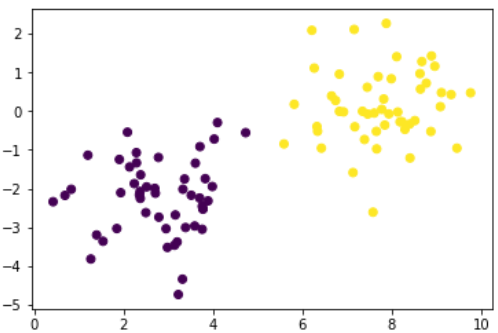f = lambda x:x + x**2 + x**1.5 +3*x**3 + x**4
a = np.linspace(0,20,100)

b = f(a)
plt.plot(a,b)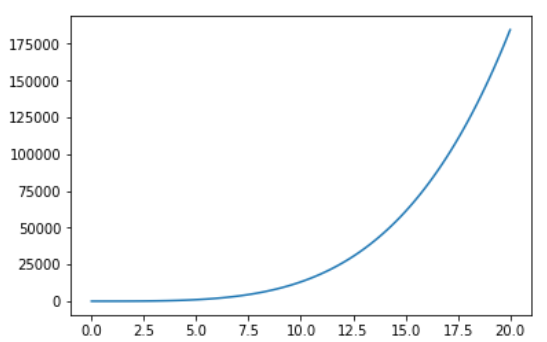# kernel 分三种：线性、rbf（基于半径，圆），ploy（多项式，绘制的图形不一样）
'''SVC(C=1.0, kernel='rbf', degree=3, gamma='auto', coef0=0.0, shrinking=True,
probability=False, tol=0.001, cache_size=200, class_weight=None, verbose=False,
max_iter=-1, decision_function_shape='ovr', random_state=None)'''
svc = SVC(kernel='linear')

svc.fit(X,y)
Out：
SVC(C=1.0, cache_size=200, class_weight=None, coef0=0.0,
decision_function_shape='ovr', degree=3, gamma='auto', kernel='linear',
max_iter=-1, probability=False, random_state=None, shrinking=True,
tol=0.001, verbose=False)

w_ = svc.coef_
w_
Out：array([[-0.39436727,  0.10620952]])

b_ = svc.intercept_
b_
Out：array([2.37576905])

# f(x,y) = w1x + w2y + b
# 三维立体中的一条直线
# f(x) = 3x + 4 f(x) = y
# y = 3x + 4
f(x,y) = z
f(x,y) = w_[0,0]*x + w_[0,1]*y + b_

z =  w_[0,0]*x + w_[0,1]*y + b_
# 当z = 0 表示，二维的平面
'''↓↓↓'''

0 =  w_[0,0]*x + w_[0,1]*y + b_

-w_[0,1]*y = w_[0,0]*x  + b_

y = -w_[0,0]/w_[0,1]*x  - b_/w_[0,1]

w = -w_[0,0]/w_[0,1]
b = - b_/w_[0,1]

display(w,b)
Out：
3.713106637047493
-22.368701893533434

plt.scatter(X[:,0],X[:,1],c = y)
x = np.linspace(0,8,100)
plt.plot(x,w*x + b,color = 'green')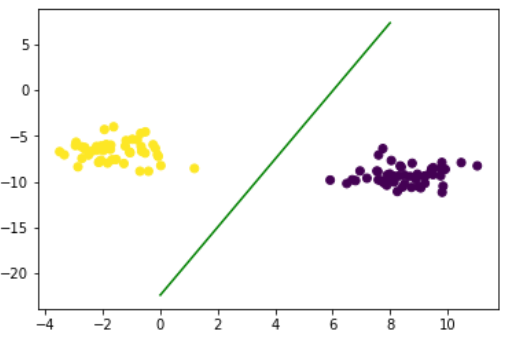supportvectors

# 支持向量，找出来的关键点
support_vectors_ = svc.support_vectors_
support_vectors_
Out：
array([[ 5.91535817, -9.81970096],
[ 1.18690432, -8.54625144]])

plt.scatter(X[:,0],X[:,1],c = y)

x = np.linspace(2,6,100)

plt.plot(x,w*x + b,color = 'green')
'''
①support_vectors_[:,0]=array([5.91535817, 1.18690432]) ,指的是x横坐标；
②s : scalar or array_like, shape (n, ), optional
size in points^2.  Default is rcParams['lines.markersize'] ** 2.
'''
plt.scatter(support_vectors_[:,0],support_vectors_[:,1],s = 300,alpha = 0.3,color = 'red')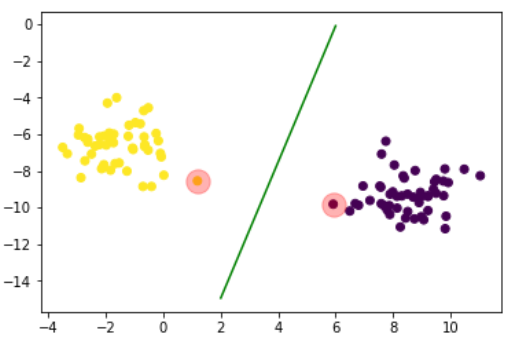# 求出两条直线，已知经过一个点（x,y），已知斜率w，求截距b：
# y = w*x + b
# b = y - w*x
'''上截距'''
b_up = support_vectors_[0,1] - w*support_vectors_[0,0]
'''下截距'''
b_down = support_vectors_[-1,1] - w*support_vectors_[-1,0]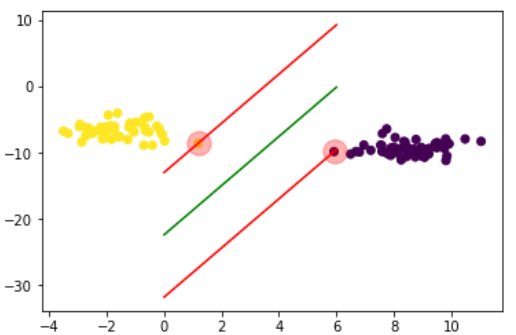3D图像：

from mpl_toolkits.mplot3d import Axes3D
fig = plt.figure(figsize=(12,9))

axes3d = Axes3D(fig)

axes3d.scatter(X[:,0],X[:,1],zs = 0)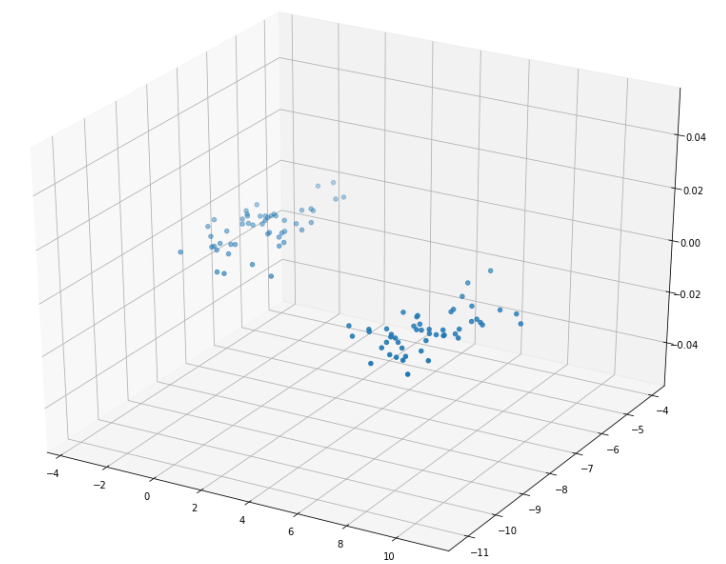### 2、SVM分离坐标点

X = np.random.randn(300,2)

plt.scatter(X[:,0],X[:,1])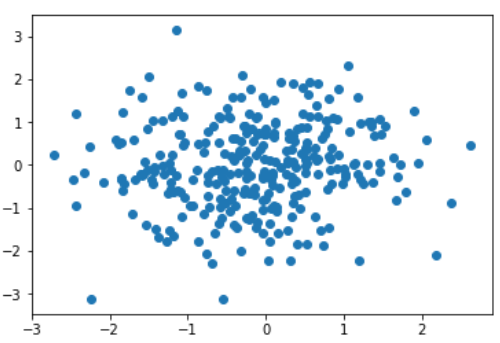# 翻看之前的逻辑运算，异或运算
y = np.logical_xor(X[:,0]>0,X[:,1]>0)
y
Out:
array([ True, False,  True, False, False, False, False, False,  True,
True,  True,  True, False, False, False,  True,  True,  True,
False, False, False, False, False,  True, False, False,  True,
False,  True,  True,  True,  True,  True, False,  True, False,
True,  True,  True, False, False,  True,  True,  True,  True,
…………
plt.figure(figsize=(8,8))
plt.scatter(X[:,0],X[:,1],c = y)

plt.axis([-3,3,-3,3])
Out：
[-3, 3, -3, 3]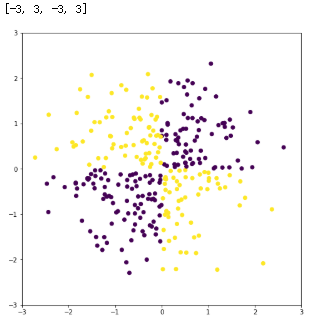a = np.linspace(-3,3,100)
b = np.linspace(-3,3,100)
A,B = np.meshgrid(a,b)

X_test = np.concatenate([A.reshape(-1,1),B.reshape(-1,1)],axis = 1)
'''plt.scatter(A,B) == plt.scatter(DataFrame(X_test),DataFrame(X_test))
== plt.scatter(X_test[:,0],X_test[:,-1])
'''
plt.scatter(A,B)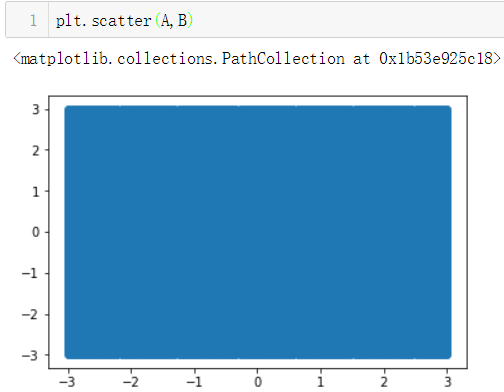svc = SVC()
svc.fit(X,y)
Out:
SVC(C=1.0, cache_size=200, class_weight=None, coef0=0.0,
decision_function_shape='ovr', degree=3, gamma='auto', kernel='rbf',
max_iter=-1, probability=False, random_state=None, shrinking=True,
tol=0.001, verbose=False)


desicion_ = svc.decision_function(X_test)
desicion_
Out:
array([-0.62425578, -0.65448087, -0.68527458, ..., -0.1871134 ,
-0.18071389, -0.17495279])
plt.figure(figsize=(8,8))

# 绘制轮廓线,等高线
# TypeError: Input must be a 2D array.
plt.contour(A,B,desicion_.reshape(100,100),cmap = 'cool')

# 绘制登高面
plt.contourf(A,B,desicion_.reshape(100,100),cmap = 'PuOr_r')

plt.scatter(X[:,0],X[:,1],c = y)

plt.axis([-3,3,-3,3])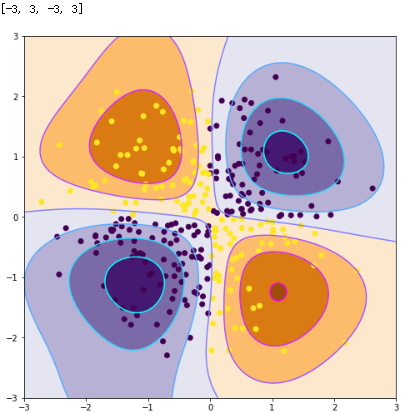### 3、使用多种核函数对iris数据集进行分类

iris = datasets.load_iris()
X = iris['data'][:,:2]
y = iris['target']

svc_linear = SVC(kernel='linear')
svc_rbf = SVC(kernel='rbf')
svc_poly = SVC(kernel='poly')

svc_linear.fit(X,y)
svc_rbf.fit(X,y)
svc_poly.fit(X,y)
Out:
SVC(C=1.0, cache_size=200, class_weight=None, coef0=0.0,
decision_function_shape='ovr', degree=3, gamma='auto', kernel='poly',
max_iter=-1, probability=False, random_state=None, shrinking=True,
tol=0.001, verbose=False)

plt.scatter(X[:,0],X[:,1],c = y)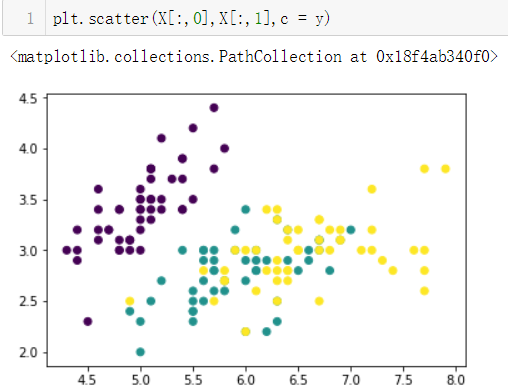a = np.linspace(4,8,300)
b = np.linspace(1.8,4.5,300)

A,B = np.meshgrid(a,b)

X_test = np.concatenate([A.reshape(-1,1),B.reshape(-1,1)],axis = 1)
linear_y_ = svc_linear.predict(X_test)

rbf_y_ = svc_rbf.predict(X_test)

poly_y_ = svc_poly.predict(X_test)

plt.figure(figsize=(12,8))

# 绘制线性
axes = plt.subplot(2,2,1)

axes.scatter(X_test[:,0],X_test[:,1],c = linear_y_)
axes.scatter(X[:,0],X[:,1],c = y,cmap = plt.cm.GnBu)

# 绘制rbf
axes = plt.subplot(2,2,2)
axes.scatter(X_test[:,0],X_test[:,1],c = rbf_y_)
axes.scatter(X[:,0],X[:,1],c = y,cmap = plt.cm.GnBu)

# 绘制多项式
axes = plt.subplot(2,2,3)
axes.scatter(X_test[:,0],X_test[:,1],c = poly_y_)
axes.scatter(X[:,0],X[:,1],c = y,cmap = plt.cm.GnBu)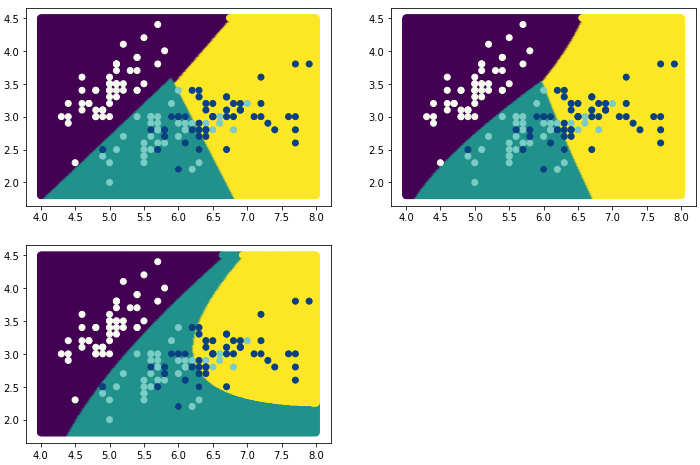### 4、使用SVM多种核函数进行回归

from sklearn.svm import SVR

import numpy as np

import matplotlib.pyplot as plt
%matplotlib inline

X = np.linspace(0,2*np.pi,40).reshape(-1,1)
y = np.sin(X)

plt.scatter(X,y)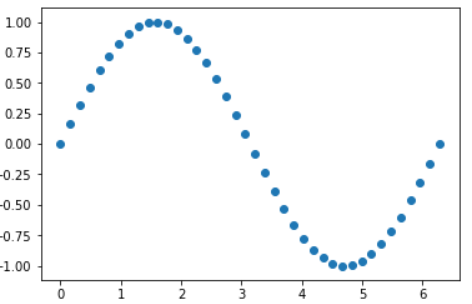y[2::5] +=np.random.randn(8,1)*0.2
plt.scatter(X,y)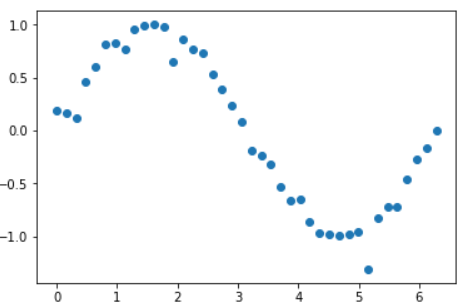svr_linear = SVR(kernel='linear')
svr_rbf = SVR(kernel='rbf')
svr_poly = SVR(kernel='poly')

svr_linear.fit(X,y)

svr_rbf.fit(X,y)

svr_poly.fit(X,y)
Out：
SVR(C=1.0, cache_size=200, coef0=0.0, degree=3, epsilon=0.1, gamma='auto',
kernel='poly', max_iter=-1, shrinking=True, tol=0.001, verbose=False)
X_test = np.linspace(0,2*np.pi,205).reshape(-1,1)
linear_y_ = svr_linear.predict(X_test)

rbf_y_ = svr_rbf.predict(X_test)

poly_y_ = svr_poly.predict(X_test)
plt.scatter(X,y)

plt.plot(X_test,linear_y_,label = 'linear')
plt.plot(X_test,rbf_y_,label = 'rbf')
plt.plot(X_test,poly_y_,label='poly')

plt.legend()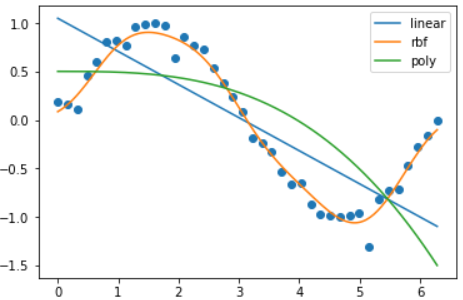12-066811

#### SVM-SVC分类

06-201万+

#### 【机器学习】svm.SVC参数详解03-14281

#### 机器学习实战(基于scikit-learn和TensorFlow)学习心得(28)--拉格朗日乘子法,多约束拉格朗日乘子法物理意义和KKT条件

12-271万+

#### sklearn.svm.SVR的参数介绍

08-278万+

#### sklearn.svm.SVC 参数说明

10-162150

#### 机器学习--支持向量机（二）拉格朗日乘子法详解

12-222229

#### 机器学习知识点总结 - 拉格朗日乘子法（Lagrange Multiplier Method）详解

11-202056

#### 【sklearn】SVM用于分类（SVC）

06-11721

#### 用PCA,LDA,KNN对MNIST数据集分类（Python）

06-20379

#### 拉格朗日乘子法与SVM分类器原理详细推导

06-302万+

#### 支持向量机SVM、支持向量回归SVR详细推导

07-124万+

#### SVM基本概要与sklearn.svm.svc 参数说明

01-275万+

#### 如何使用sklearn中的SVM

06-2099

#### 今日头条秋招开启，帮助解析面试题准备数据分析岗位

12-281263

#### sklearn中的svm.SVC

07-182416

#### 软件测试入门、SQL、性能测试、测试管理工具

03-02©️2020 CSDN 皮肤主题: 技术黑板 设计师: CSDN官方博客点击重新获取扫码支付1.余额是钱包充值的虚拟货币，按照1:1的比例进行支付金额的抵扣。
2.余额无法直接购买下载，可以购买VIP、C币套餐、付费专栏及课程。余额充值